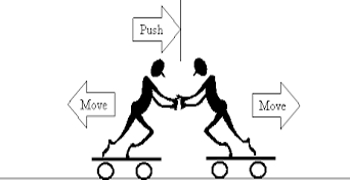# Newton’s Third Law of Motion

Newton’s Third Law of Motion states that ‘To every action there is an equal and opposite reaction’.

It is a common observation that when we sit on a chair, our body exerts a downward force on the chair and the chair exerts an upward force on our body. There are two forces resulting from this interaction: a force on the chair and a force on our body. These two forces are called action and reaction forces. Newton’s third law explains the relation between these action forces. It states that for every action, there is an equal and opposite reaction.

Whenever one body exerts a certain force on a second body, the second body exerts an equal and opposite force on the first. Newton’s third law is sometimes called as the law of action and reaction.

Let there be two bodies 1 and 2 exerting the force exerted on forces on each other. Let the force exerted on the body 1by the body 2 be F12 and the force exerted on the body 2 by the body 1 be F21. Then accordind to third law. F12 = – F21One of these forces, say F12 may be called as the action whereas the other force F21 may be called as the reaction or vice versa. This implies that we cannot say which is the cause (action) or which is the effect (reaction). It is to be noted that always the action and reaction do not act on the same body; they always act on different bodies. The action and reaction never cancel each other and the forces always exist in pair.

The effect of third law of motion can be observed in many activities in our everyday life.

The examples are:

(i) When a bullet is fired from a gun with a certain force (action), there is an equal and opposite force exerted on the gun in the backward direction (reaction).

(ii) When a man jumps from a boat to the shore, the boat moves away from him. The force he exerts on the boat (action) is responsible for its motion and his motion to the shore is due to the force of reaction exerted by the boat on him.

(iii) The swimmer pushes the water in the backward direction with a certain force (action) and the water pushes the swimmer in the forward direction with an equal and opposite force (reaction).